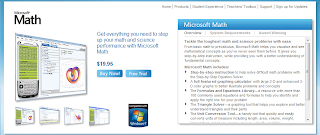### Microsoft Maths : Tackle the toughest Math and Science problems with easeFrom basic math to precalculus, Microsoft Math helps you visualize and see mathematical concepts as you’ve never seen them before. It gives you step-by-step instruction, while providing you with a better understanding of fundamental concepts.

Microsoft Math includes:
• Step-by-step instruction to help solve difficult math problems with the Step-by-Step Equation Solver.
• A full-featured graphing calculator with large 2-D and enhanced 3-D color graphs to better illustrate problems and concepts
• The Formulas and Equations Library—a resource with more than 100 commonly used equations and formulas to help you identify and apply the right one for your problem
• The Triangle Solver—a graphing tool that helps you explore and better understand triangles and their parts
• The Unit Conversion Tool—a handy tool that quickly and easily converts units of measure including length, area, volume, weight, temperature, pressure, energy, power, velocity, and time.
• Ink Handwriting Support that works with Tablet PCs and Ultra-Mobile PCs and recognizes handwritten problems.